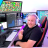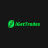# Looking for Parabolic SAR MTF (Multi Time Frame)

F

#### flyer01

##### New member
Would anyone be willing to share code for a MTF PSAR script. For example, on a 1 hour chart I would like to see the Parabolic SAR plotted from a 4 hour aggregation. Is this possible?#### horserider

##### Well-known member
VIP
@flyer01 Here is 4 hour agg. Can input agg of your choice to display PSAR at a different agg than chart.

Code:
``````#
# TD Ameritrade IP Company, Inc. (c) 2008-2019 ParabolicSAR
# Changed to 4 hour aggregation by Horserider 12/13/2019

input accelerationFactor = 0.02;
input accelerationLimit = 0.2;

Assert(accelerationFactor > 0, "'acceleration factor' must be positive: " + accelerationFactor);
Assert(accelerationLimit >= accelerationFactor, "'acceleration limit' (" + accelerationLimit + ") must be greater than or equal to 'acceleration factor' (" + accelerationFactor + ")");

def state = {default init, long, short};
def extreme;
def SAR;
def acc;
input agg = AggregationPeriod.FOUR_HOURS;
def high = high (period = agg);
def low = low (period = agg);
switch (state) {
case init:
state = state.long;
acc = accelerationFactor;
extreme = high;
SAR = low;
case short:
if (SAR < high)
then {
state = state.long;
acc = accelerationFactor;
extreme = high;
SAR = extreme;
} else {
state = state.short;
if (low < extreme)
then {
acc = Min(acc + accelerationFactor, accelerationLimit);
extreme = low;
} else {
acc = acc;
extreme = extreme;
}
SAR = Max(Max(high, high), SAR + acc * (extreme - SAR));
}
case long:
if (SAR > low)
then {
state = state.short;
acc = accelerationFactor;
extreme = low;
SAR = extreme;
} else {
state = state.long;
if (high > extreme)
then {
acc = Min(acc + accelerationFactor, accelerationLimit);
extreme = high;
} else {
acc = acc;
extreme = extreme;
}
SAR = Min(Min(low, low), SAR + acc * (extreme - SAR));
}
}

plot parSAR = SAR;
parSAR.SetPaintingStrategy(PaintingStrategy.POINTS);
parSAR.SetDefaultColor(GetColor(5));``````

•SolidChiken
F

#### flyer01

##### New member
@horserider, that is exactly what I was looking for. Thank you so much!

S

#### stockminion

##### New member
VIP
Can we make this work in Mobile @horserider .#### BenTen

Staff
VIP
@stockminion MTF doesn’t work in the mobile app.

S

VIP
Nps. Thanks.

D

#### Dazeinhjk

##### New member
VIP
@flyer01 Here is 4 hour agg. Can input agg of your choice to display PSAR at a different agg than chart.

Code:
``````#
# TD Ameritrade IP Company, Inc. (c) 2008-2019 ParabolicSAR
# Changed to 4 hour aggregation by Horserider 12/13/2019

input accelerationFactor = 0.02;
input accelerationLimit = 0.2;

Assert(accelerationFactor > 0, "'acceleration factor' must be positive: " + accelerationFactor);
Assert(accelerationLimit >= accelerationFactor, "'acceleration limit' (" + accelerationLimit + ") must be greater than or equal to 'acceleration factor' (" + accelerationFactor + ")");

def state = {default init, long, short};
def extreme;
def SAR;
def acc;
input agg = AggregationPeriod.FOUR_HOURS;
def high = high (period = agg);
def low = low (period = agg);
switch (state) {
case init:
state = state.long;
acc = accelerationFactor;
extreme = high;
SAR = low;
case short:
if (SAR < high)
then {
state = state.long;
acc = accelerationFactor;
extreme = high;
SAR = extreme;
} else {
state = state.short;
if (low < extreme)
then {
acc = Min(acc + accelerationFactor, accelerationLimit);
extreme = low;
} else {
acc = acc;
extreme = extreme;
}
SAR = Max(Max(high, high), SAR + acc * (extreme - SAR));
}
case long:
if (SAR > low)
then {
state = state.short;
acc = accelerationFactor;
extreme = low;
SAR = extreme;
} else {
state = state.long;
if (high > extreme)
then {
acc = Min(acc + accelerationFactor, accelerationLimit);
extreme = high;
} else {
acc = acc;
extreme = extreme;
}
SAR = Min(Min(low, low), SAR + acc * (extreme - SAR));
}
}

plot parSAR = SAR;
parSAR.SetPaintingStrategy(PaintingStrategy.POINTS);
parSAR.SetDefaultColor(GetColor(5));``````

Nice study. Im using, is so useful. Do you know any study with a Parabolic SAR but crossover MTF? Im looking an arrow to see the trend within parabolicSAR. For example, in lower timeframe I would like to know when the price crossover parabolic in higher timeframe.Looking for average price position line upper study indicators Questions 3Looking for Gap Up Scanner with EMA's Questions 1